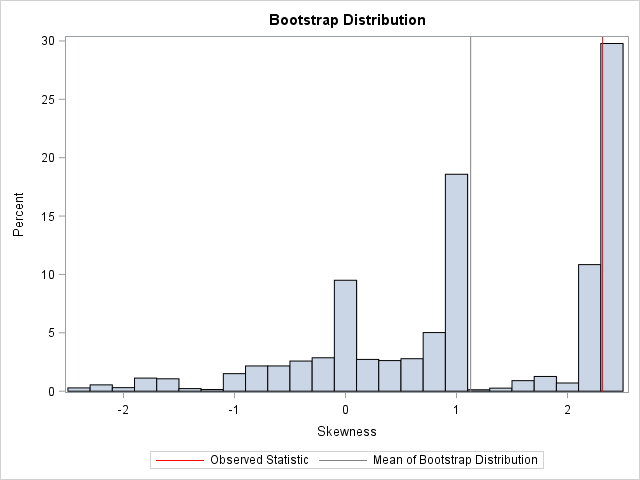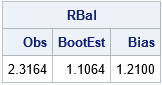This article shows how to implement balanced bootstrap sampling in SAS. The basic bootstrap samples with replacement from the original data (N observations) to obtain B new samples. This is called "uniform" resampling because each observation has a uniform probability of 1/N of being selected at each step of the resampling process. Within the union of the B bootstrap samples, each observation has an expected value of appearing B times.

Balanced bootstrap resampling (Davison, Hinkley, and Schechtman, 1986) is an alternative process in which each observation appears exactly B times in the union of the B bootstrap samples of size N. This has some practical benefits for estimating certain inferential statistics such as the bias and quantiles of the sampling distribution (Hall, 1990).

It is easy to implement a balanced bootstrap resampling scheme: Concatenate B copies of the data, randomly permute the B*N observations, and then use the first N observations for the first bootstrap sample, the next B for the second sample, and so forth. (Other algorithms are also possible, as discussed by Gleason, 1988). This article shows how to implement balanced bootstrap sampling in SAS.

### Balanced bootstrap samples in SAS

To illustrate the idea, consider the following data set that has N=6 observations. Five observations are clustered near x=0 and the sixth is a large outlier (x=10). The sample skewness for these data is skew=2.316 because of the influence of the outlier.

```data Sample(keep=x); input x @@; datalines; -1 -0.2 0 0.2 1 10 ;   proc means data=Sample skewness; run; %let ObsStat = 2.3163714;```

You can use the bootstrap to approximate the sampling distribution for the skewness statistic for these data. I have previously shown how to use SAS to bootstrap the skewness statistic: Use PROC SURVEYSELECT to form bootstrap samples, use PROC MEANS with a BY statement to analyze the samples, and use PROC UNIVARIATE to analyze the bootstrap distribution of skewness values. In that previous article, PROC SURVEYSELECT is used to perform uniform sampling (sampling with replacement).

It is straightforward to modify the previous program to perform balanced bootstrap sampling. The following program is based on a SAS paper by Nils Penard at PhUSE 2012. It does the following:

1. Use PROC SURVEYSEELCT to concatenate B copies of the input data.
2. Use the DATA step to generate a uniform random number for each observation.
3. Use PROC SORT to sort the data by the random values. After this step, the N*B observations are in random order.
4. Generate a variable that indicates the bootstrap sample for each observation. Alternatively, reuse the REPLICATE variable from PROC SURVEYSELECT, as shown below.
```/* balanced bootstrap computation */ proc surveyselect data=Sample out=DupData noprint reps=5000 /* duplicate data B times */ method=SRS samprate=1; /* sample w/o replacement */ run;   data Permute; set DupData; call streaminit(12345); u = rand("uniform"); /* generate a uniform random number for each obs */ run;   proc sort data=Permute; by u; run; /* sort in random order */   data BalancedBoot; merge DupData(drop=x) Permute(keep=x); /* reuse REPLICATE variable */ run;```

You can use the BalancedBoot data set to perform subsequent bootstrap analyses. If you perform a bootstrap analysis, you obtain the following approximate bootstrap distribution for the skewness statistic. The observed statistic is indicated by a red vertical line. For reference, the mean of the bootstrap distribution is indicated by a gray vertical line. You can see that the sampling distribution for this tiny data set is highly nonnormal. Many bootstrap samples that contain the outlier (exactly one-sixth of the samples in a balanced bootstrap) will have a large skewness value.To assure yourself that each of the original six observations appears exactly B times in the union of the bootstrap sample, you can run PROC FREQ, as follows:

```proc freq data=BalancedBoot; /* OPTIONAL: Show that each obs appears B times */ tables x / nocum; run;```

### Balanced bootstrap samples in SAS/IML

As shown in the article "Bootstrap estimates in SAS/IML," you can perform bootstrap computations in the SAS/IML language. For uniform sampling, the SAMPLE function samples with replacement from the original data. However, you can modify the sampling scheme to support balanced bootstrap resampling:

1. Use the REPEAT function to duplicate the data B times.
2. Use the SAMPLE function with the "WOR" option to sample without replacement. The resulting vector is a permutation of the B*N observations.
3. Use the SHAPE function to reshape the permuted data into an N x B matrix for which each column is a bootstrap sample. This form is useful for implementing vectorized computations on the columns.

The following SAS/IML program modifies the program in the previous post to perform balanced bootstrap sampling:

```/* balanced bootstrap computation in SAS/IML */ proc iml; use Sample; read all var "x"; close; call randseed(12345);   /* Return a row vector of statistics, one for each column. */ start EvalStat(M); return skewness(M); /* <== put your computation here */ finish; Est = EvalStat(x); /* 1. observed statistic for data */   /* balanced bootstrap resampling */ B = 5000; /* B = number of bootstrap samples */ allX = repeat(x, B); /* replicate the data B times */ s = sample(allX, nrow(allX), "WOR"); /* 2. sample without replacement (=permute) */ s = shape(s, nrow(x), B); /* reshape to (N x B) */   /* use the balanced bootstrap samples in subsequent computations */ bStat = T( EvalStat(s) ); /* 3. compute the statistic for each bootstrap sample */ bootEst = mean(bStat); /* 4. summarize bootstrap distrib such as mean */ bias = Est - bootEst; /* Estimate of bias */ RBal = Est || BootEst || Bias; /* combine results for printing */ print RBal[format=8.4 c={"Obs" "BootEst" "Bias"}];```As shown in the previous histogram, the bias estimate (the difference between the observed statistic and the mean of the bootstrap distribution) is sizeable.

It is worth mentioning that the SAS-supplied %BOOT macro performs balanced bootstrap sampling by default. To generate balanced bootstrap samples with the %BOOT macro, set the BALANCED=1 option, as follows:
%boot(data=Sample, samples=5000, balanced=1) /* or omit BALANCED= option */
If you want uniform (unbalanced) samples, call the macro as follows:
%boot(data=Sample, samples=5000, balanced=0).

In conclusion, it is easy to generate balanced bootstrap samples. Balanced sampling can improve the efficiency of certain bootstrap estimates and inferences. For details, see the previous references of Appendix II of Hall (1992).

Share